# Get the Hour from timestamp in Pandas

Let’s see how to extract the hour from a timestamp in Pandas, with the help of multiple examples.

Example 1 : `pandas.timestamp.now()` takes timezone as input and returns current timestamp object of that timezone.

 `# importing the module ` `import` `pandas as pd ` ` `  `# input current timestamp ` `date ``=` `pd.Timestamp.now() ` `print``(``"currentTimestamp: "``, date) ` ` `  `# extract the Hours from the timestamp ` `frame ``=` `date.hour ` `print``(``"Hour: "``, frame) `

Output: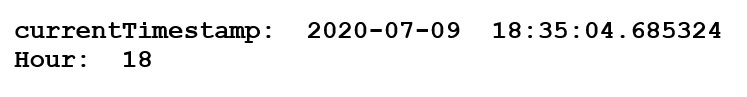Example 2 : `pandas.timestamp()` is used for DateTimeIndex of a specific timezone. It takes year, month, day, time and timezone as input and returns DateTimeIndex of that timezone.

 `# importing the module ` `import` `pandas as pd    ` ` `  `# input the timestamp ` `date ``=` `pd.Timestamp(year ``=` `2020``, month ``=` `7``, day ``=` `21``,  ` `                    ``hour ``=` `6``, minute ``=` `30``, second ``=` `44``,  ` `                    ``tz ``=` `'Asia / Kolkata'``)   ` `print``(``"Timestamp: "``, date) ` ` `  `# extract the Hours from the timestamp ` `print``(``"Hour: "``, date.hour) `

Output :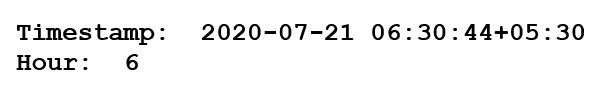Example 3 : Take input as range of timestamps using `pandas.dt_range()` and use `pandas.series()` to convert into an array of timestamps.

 `# importing the module ` `import` `pandas as pd  ` ` `  `# take input Dates in a range ` `dates ``=` `pd.Series(pd.date_range(``'2019-8-5 10:23:05'``, periods ``=` `6``, freq ``=``'H'``)) ` ` `  `# convert in a dict container ` `frame ``=` `pd.DataFrame(``dict``(givenDate ``=` `dates)) ` ` `  `# extract Hours from Timestamp ` `frame[``'hourOfTimestamp'``] ``=` `frame[``'givenDate'``].dt.hour ` `print``(frame) `

Output :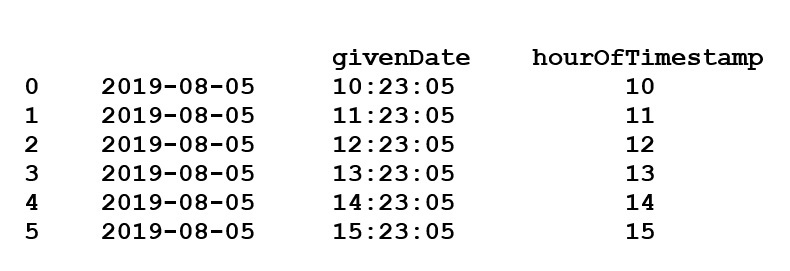Solution 4 : Use `object.hour` attribute to return the hour of the datetime within data of the given Series object.

 `# importing the module ` `import` `pandas as pd  ` ` `  `# take inputs ` `dates ``=` `pd.Series([``'2015-01-11 09:20'``, ``'2019-4-8 11:31'``, ``'2018-12-22 10:10'``,  ` `                   ``'2011-4-2 04:25'``, ``'2017-1-6 03:51'``])   ` ` `  `# give a Name to the series ` `seriesName ``=` `[``'T1'``, ``'T2'``, ``'T3'``, ``'T4'``, ``'T5'``]   ` ` `  `# give index to each timestamp ` `dates.index ``=` `seriesName ` ` `  `dates ``=` `pd.to_datetime(dates) ` ` `  `# extract Hours from Timestamp  ` `rs ``=` `dates.dt.hour   ` `print``(rs) `

Output :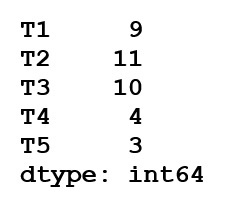Solution 5 : Read timestamp data from a csv file and get Hours from each timestamp.

 `# importing the module ` `import` `pandas as pd ` ` `  `# read the date from xyz.csv file ` `frame ``=` `pd.read_csv(r``'xyz.csv'``) ` `print``(``"Values in xyz.csv: "``) ` `print``(frame.head()) ` ` `  `frame[``'dateTime'``] ``=` `frame[``'dateTime'``].astype(``'datetime64[ns]'``) ` ` `  `# extract Hours from Timestamp   ` `print``(``"Hours: "``) ` `print``(frame.dateTime.dt.hour.head()) `

Output :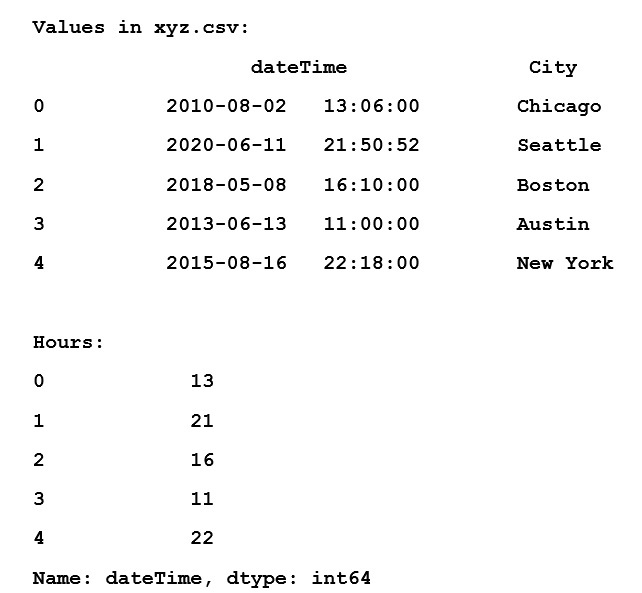Attention geek! Strengthen your foundations with the Python Programming Foundation Course and learn the basics.

To begin with, your interview preparations Enhance your Data Structures concepts with the Python DS Course.

My Personal Notes arrow_drop_upCheck out this Author's contributed articles.

If you like GeeksforGeeks and would like to contribute, you can also write an article using contribute.geeksforgeeks.org or mail your article to contribute@geeksforgeeks.org. See your article appearing on the GeeksforGeeks main page and help other Geeks.

Please Improve this article if you find anything incorrect by clicking on the "Improve Article" button below.

Article Tags :

Be the First to upvote.

Please write to us at contribute@geeksforgeeks.org to report any issue with the above content.